• ## 高斯核函数

万次阅读 多人点赞 2018-03-21 17:08:15
线性支持向量机 (Linear-...高斯核函数（Gaussian kernel），也称径向基 (RBF) 函数，是常用的一种核函数。它可以将有限维数据映射到高维空间，我们来看一下高斯核函数的定义： k(x,x′)=e−||x−x′||22σ2k(x, x')
线性支持向量机 (Linear-SVM) 被用于线性可分的数据集的二分类问题，当数据集不是线性可分的时候，需要利用到核函数将数据集映射到高维空间。这样数据在高维空间中就线性可分。

高斯核函数（Gaussian kernel），也称径向基 (RBF) 函数，是常用的一种核函数。它可以将有限维数据映射到高维空间，我们来看一下高斯核函数的定义： k(x,x′)=e−||x−x′||22σ2<!--//--><![CDATA[//><!--
k(x, x') = e^{-\frac{||x - x'||^2}{2\sigma^2}}
//--><!]]>
上述公式涉及到两个向量的欧式距离（2范数）计算，而且，高斯核函数是两个向量欧式距离的单调函数。σ<!--//--><![CDATA[//><!--
\sigma
//--><!]]> 是带宽，控制径向作用范围，换句话说，σ<!--//--><![CDATA[//><!--
\sigma
//--><!]]> 控制高斯核函数的局部作用范围。当x<!--//--><![CDATA[//><!--
x
//--><!]]> 和x′<!--//--><![CDATA[//><!--
x'
//--><!]]> 的欧式距离处于某一个区间范围内的时候，假设固定x′<!--//--><![CDATA[//><!--
x'
//--><!]]>，k(x,x′)<!--//--><![CDATA[//><!--
k(x, x')
//--><!]]> 随x的变化而变化的相当显著。

一维情况

令x′=0<!--//--><![CDATA[//><!--
x'  = 0
//--><!]]>，k(x,0)<!--//--><![CDATA[//><!--
k(x, 0)
//--><!]]> 随x的变化情况如下图所示：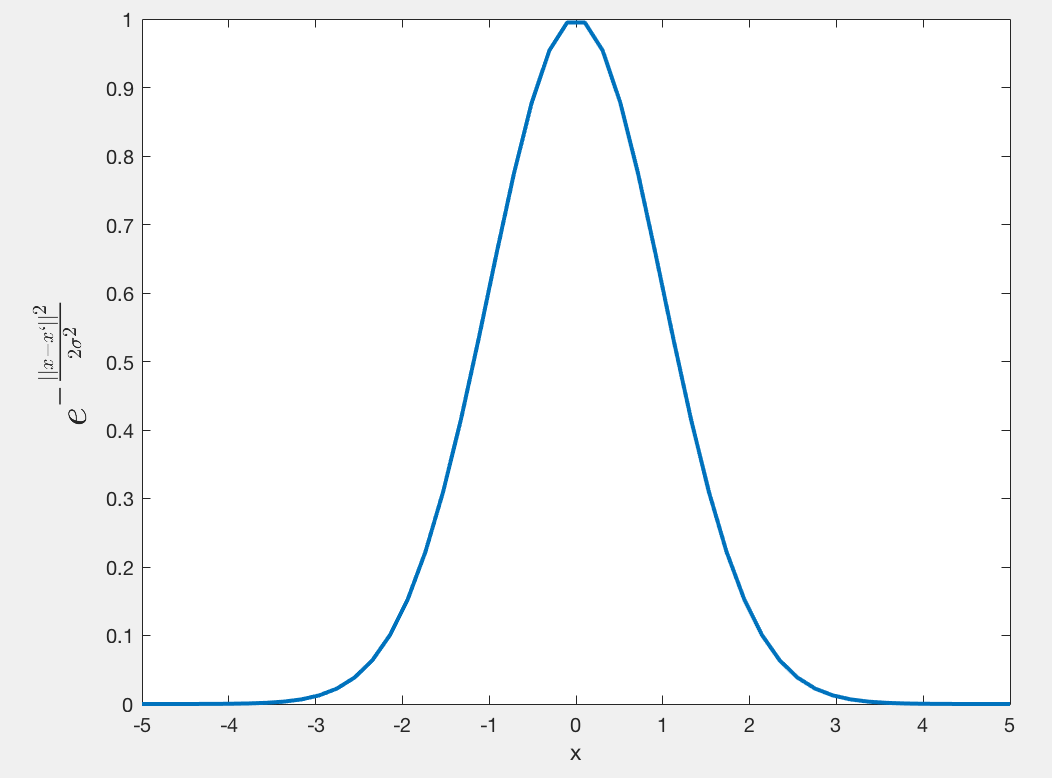σ=1<!--//--><![CDATA[//><!--
\sigma = 1
//--><!]]>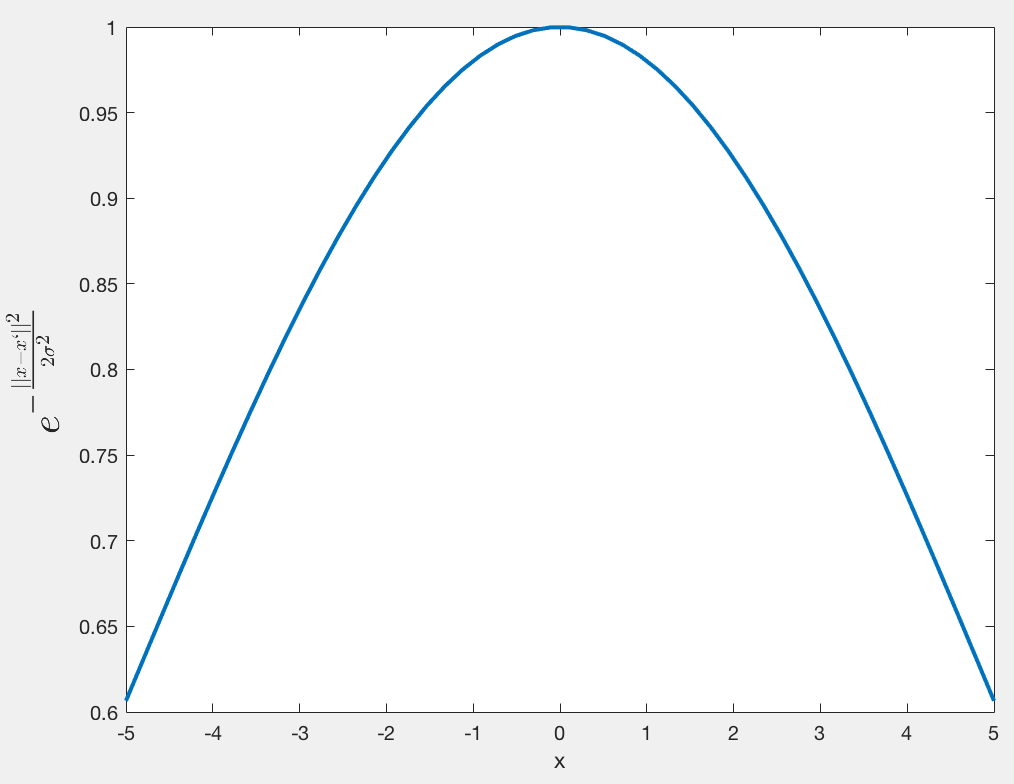σ=5<!--//--><![CDATA[//><!--
\sigma = 5
//--><!]]>
我们看到，随着x<!--//--><![CDATA[//><!--
x
//--><!]]> 与x′<!--//--><![CDATA[//><!--
x'
//--><!]]>的距离的距离的增大，其高斯核函数值在单调递减。并且，σ<!--//--><![CDATA[//><!--
\sigma
//--><!]]>越大，那么高斯核函数的局部影响范围就会越大。

二维情况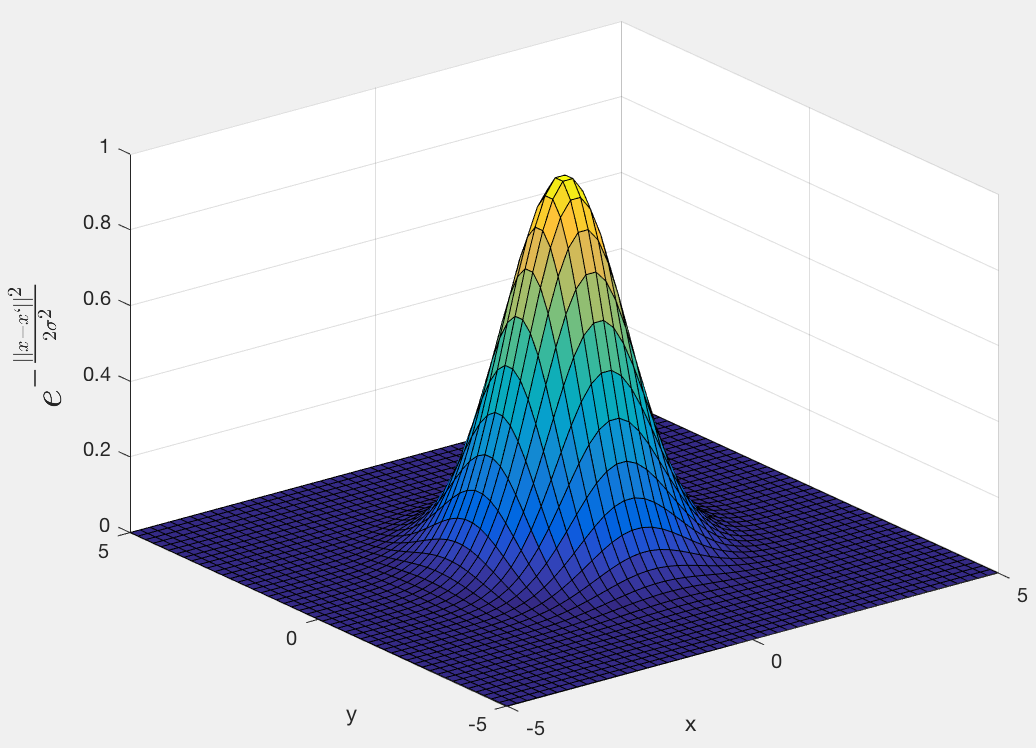σ=1<!--//--><![CDATA[//><!--
\sigma=1
//--><!]]>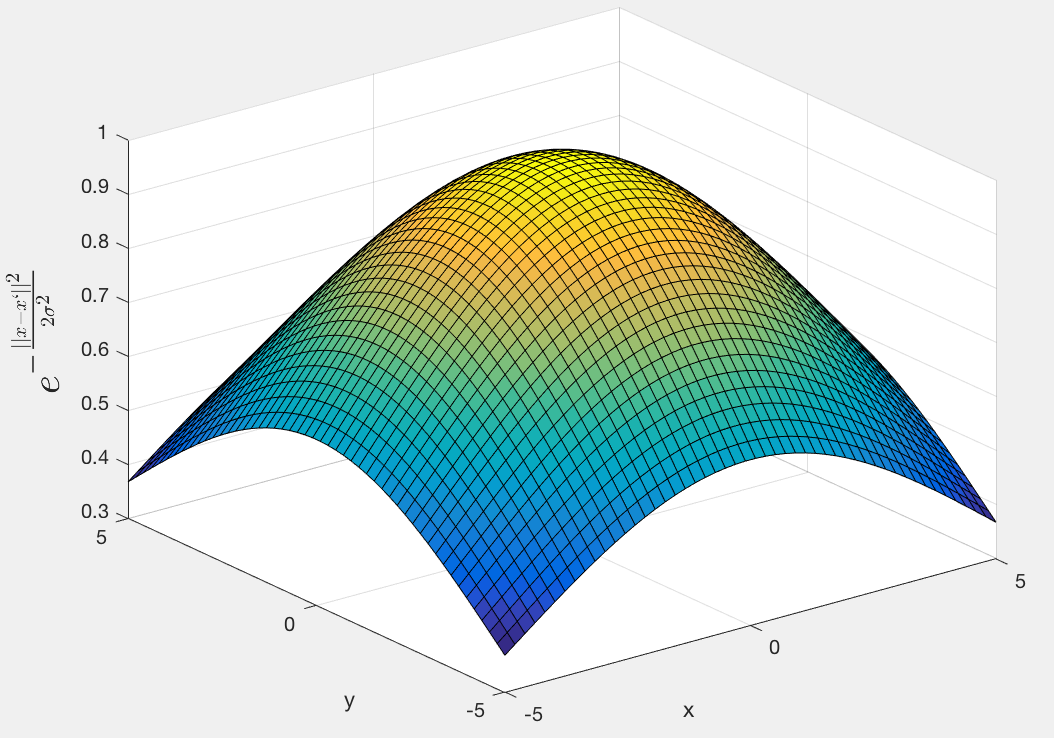σ=5<!--//--><![CDATA[//><!--
\sigma=5
//--><!]]>

二维可以更加明显的看出高斯核函数局部作用的范围随带通的变化情况。带通越大，高斯核函数的局部影响的范围就越大。在超出这个范围之后，核函数的值几乎不变。

高斯核将数据映射到高维甚至无穷维的原理

通过一些简单的推导，我们可以得到这样的结果，为了描述简单，我们令高斯核中的分母为1.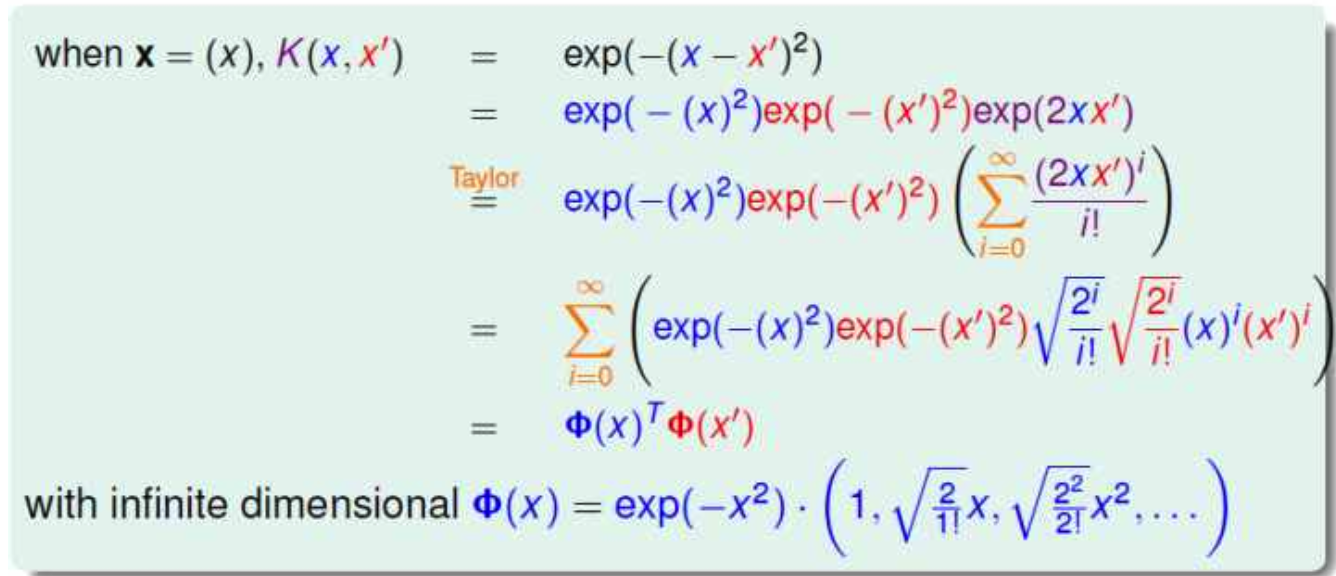图片转载自：https://www.zhihu.com/question/46587416?from=profile_question_card
可以看到，高斯核函数通过泰勒展开可以被描述成ϕ(x)Tϕ(x′)<!--//--><![CDATA[//><!--
\phi(x)^T\phi(x')
//--><!]]>的形式，而ϕ(x)<!--//--><![CDATA[//><!--
\phi(x)
//--><!]]>是无穷维的。
展开全文kernel SVM
• opencv实现高斯核函数。 1)sigma可控的高斯核函数 2）sigma和维度可控的高斯核函数
• 这篇博客主要学习一种特殊的核函数，而且它也是 SVM 算法使用最多的一种核函数：高斯核函数。 核函数我们通常表示成： 那么对于高斯核函数来说，定义方式如下： 其中只有一个超参数 γγγ。那么为什么这样的一个...
高斯核函数
上一篇博客详细的介绍了什么是核函数，并且主要以多项式核函数为例。这篇博客主要学习一种特殊的核函数，而且它也是 SVM 算法使用最多的一种核函数：高斯核函数。
核函数我们通常表示成：那么对于高斯核函数来说，定义方式如下：其中只有一个超参数 $γ$。那么为什么这样的一个核函数要称做高斯核函数呢？

相信很多小伙伴都听过正态分布，其实正态分布就是一个高斯函数。其实我们仔细观察高斯核函数与正态分布之间形式大体是一致的。高斯核函数有时候又被称做 RBF核（Radial Basis Function Kernel），中文有时候译为镜像基函数。高斯核函数的本质是将每一个样本点映射到一个无穷维的特征空间，听起来非常的抽象。

下面我尝试用一个简单的例子来模拟一下高斯核函数到底在做什么事情？

先回忆一下使用多项式特征为什么可以处理非线性的数据问题，那么它的基本原理其实是依靠升维使得原本线性不可分的数据变得线性可分。

比如下面有一些一维数据就放在了一个坐标轴上，我们现在是没法用一条直线将这些样本点分成两类。但是如果我们添加上多项式特征，就是让每一个数据点不但有 $x$ 值，还有一个纵轴，有一个第二个维度的值，该例子中就让第二个维度的值为 $x^2$，那么我们所有的数据点就变成了：这些数据点在 $x$ 轴上的位置是没有变的，只不过在 $y$ 轴上也有一个取值，这个取值就是 $x^2$，现在这些数据点原来在一维空间中是线性不可分的，现在到了二维空间，就变得线性可分了。其实高斯核本质上做的事情也是这样的。高斯核函数如下：在这里，为了可视化方便，将高斯核函数的式子中的 $y$ 取固定的两个点 $l1$，$l2$（称为地标）。现在我们的数据依然是线性不可分的。高斯核就将原本一维的样本 $x$ 映射为一个二维（有几个地标，就映射为几维）的数据点：首先我们用程序来模拟高斯核的映射过程。很显然，上图是线性可分的，我们很容易找到一条直线，将这些样本点分成两类：但是实际，我们的高斯核函数是这样的：在这个 $y$ 的位置，其实是每一个数据点。换句话说，高斯核做的事情和之前做的事情一样，只不过取了更多的地标点而已，我们有多少个样本就有多少个地标点。  换句话说，对于每一个 $x$，都要尝试对每一个样本 $y$ 进行核函数的计算成为新的高维数据所对应的某一个维度的元素。所以高斯核函数就是将 $m*n$ 的数据映射成了 $m*m$ 的数据。
具体代码见 76 高斯核函数.ipynb


展开全文• 这种回归方式称为高斯核函数回归。依据不同形式的核函数，可以计算出不同的自变量样本权重。 期权的隐含波动率与期权的在值程度和期权剩余到期时间具有函数关系。本文用高斯核函数回归的方式，选取2020年12月30日...
假设自变量为X，因变量为Y。X中含有n个样本，并且与Y中的值一一对应。对于自变量样本的任意取值x，它有个一唯一对应的y。为了计算y，利用其他自变量样本对应的y取加权平均值，权重为其他自变量样本距离该x的样本距离。距离x越远的样本，则对应的y的权重越小。这种回归方式称为高斯核函数回归。依据不同形式的核函数，可以计算出不同的自变量样本权重。
期权的隐含波动率与期权的在值程度和期权剩余到期时间具有函数关系。本文用高斯核函数回归的方式，选取2020年12月30日上证50ETF期权的数据，建立隐含波动率模型。样本数据见：
1.导入模块和不同的核函数
from sklearn.datasets import make_friedman2
from sklearn.gaussian_process import GaussianProcessRegressor
"""用于引入核的形式https://scikit-learn.org/stable/modules/gaussian_process.html#rw2006
ConstantKernel:修改高斯过程的均值
WhiteKernel:修改高斯过程的标准差
Sum:将两核求和
Product:将两核求积
Exponentiation:将核求指数
RBF：径向基函数(RBF)核
Matern：Matern内核
RationalQuadratic:二次有理核
ExpSineSquared:指数sin平方核
DotProduct：点乘核
"""
from sklearn.gaussian_process.kernels import *
import pandas as pd
from sklearn import preprocessing
from sklearn.model_selection import train_test_split
from sklearn.metrics import *#模型评估指标

2.处理样本数据
#读取数据
data=pd.read_excel('隐含波动率样本数据.xlsx')
X=data[['times', 'm']]
y=data['implied']

#将自变量做标准化处理
X=preprocessing.scale(X)

#将数据划分为训练集和测试集
x_train,x_test,y_train,y_test=train_test_split(X,y,test_size=0.2,random_state=12)

3.拟合模型
kernel = Matern(length_scale=0.484,nu=1.5) + WhiteKernel()#构造核函数形式

model= GaussianProcessRegressor(kernel=kernel,#指定GP协方差函数的内核
alpha=1e-10,#拟合时核矩阵对角线上增加的值.通过确保计算值形成一个正定矩阵，可以防止在拟合过程中出现潜在的数值问题
optimizer='fmin_l_bfgs_b',#用于优化内核参数的内部支持的优化器
n_restarts_optimizer=0,#优化器重新启动的次数，用于寻找最大化对数边际可能性的内核参数
normalize_y=False,#不管目标值y是否归一化，目标值的均值和方差分别设为0和1
copy_X_train=True,
random_state=None)
model=model.fit(X, y)#拟合高斯过程回归模型

4.观察模型
model.get_params()#获取这个估计量的参数
model.log_marginal_likelihood()#为训练数据返回theta的对数边际可能性
model.score(X, y)#返回拟合优度
model.sample_y([[-0.63252516,  0.8778663 ]])#从高斯过程中抽取样本，在X处求值
model.predict(X, return_std=True)#使用高斯过程回归模型进行预测

5.评估模型拟合效果
y_pred=model.predict(x_test)#测试集数据的预测值
explained_variance_score(y_true=y_test,y_pred=y_pred)#解释方差分数
mean_absolute_error(y_true=y_test,y_pred=y_pred)#绝对误差均值
mean_absolute_percentage_error(y_true=y_test,y_pred=y_pred)#绝对误差百分比
mean_squared_error(y_true=y_test,y_pred=y_pred)#均方误差
mean_squared_log_error(y_true=y_test,y_pred=y_pred)#均方对数误差
r2_score(y_true=y_test,y_pred=y_pred)#拟合优度



展开全文• 机器学习：SVM（核函数、高斯核函数RBF） 一、核函数（Kernel Function） 　1）格式 K(x, y)：表示样本 x 和 y，添加多项式特征得到新的样本 x'、y'，K(x, y) 就是返回新的样本经过计算得到的值； 在 SVM 类型的...
转载自博客园：https://www.cnblogs.com/volcao/p/9465214.html

SVM（核函数、高斯核函数RBF）

一、核函数（Kernel Function）

1）格式

K(x, y)：表示样本 x 和 y，添加多项式特征得到新的样本 x'、y'，K(x, y) 就是返回新的样本经过计算得到的值；
在 SVM 类型的算法 SVC() 中，K(x, y) 返回点乘：x' . y' 得到的值；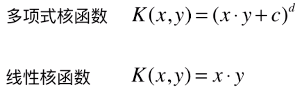2）多项式核函数

业务问题：怎么分类非线性可分的样本的分类？

内部实现：

对传入的样本数据点添加多项式项；
新的样本数据点进行点乘，返回点乘结果；
多项式特征的基本原理：依靠升维使得原本线性不可分的数据线性可分；
升维的意义：使得原本线性不可分的数据线性可分；

例：

一维特征的样本，两种类型，分布如图，线性不可分：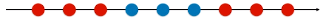为样本添加一个特征：x2 ，使得样本在二维平面内分布，此时样本在 x 轴升的分布位置不变；如图，可以线性可分：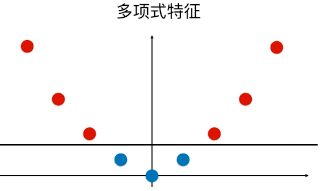3）优点 / 特点

不需要每次都具体计算出原始样本点映射的新的无穷维度的样本点，直接使用映射后的新的样本点的点乘计算公式即可；
减少计算量
减少存储空间
一般将原始样本变形，通常是将低维的样本数据变为高维数据，存储高维数据花费较多的存储空间；使用核函数，不用考虑原来样本改变后的样子，也不用存储变化后的结果，只需要直接使用变化的结果进行运算并返回运算结果即可；
核函数的方法和思路不是 SVM 算法特有，只要可以减少计算量和存储空间，都可以设计核函数方便运算；
对于比较传统的常用的机器学习算法，核函数这种技巧更多的在 SVM 算法中使用；
4）SVM 中的核函数

svm 类中的 SVC() 算法中包含两种核函数：
SVC(kernel = 'ploy')：表示算法使用多项式核函数；
SVC(kernel = 'rbf')：表示算法使用高斯核函数；
SVM 算法的本质就是求解目标函数的最优化问题；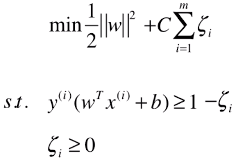求解最优化问题时，将数学模型变形：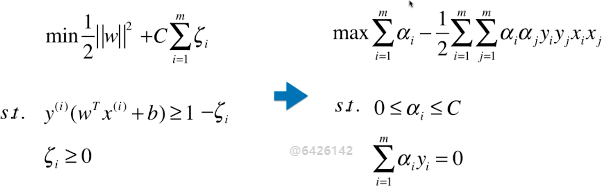5）多项式核函数

格式：

from sklearn.svm import SVC

svc = SVC(kernel = 'ploy')

思路：设计一个函数（ K(xi, xj) ），传入原始样本（x(i) 、 x(j)），返回添加了多项式特征后的新样本的计算结果（x'(i) . x'(j)）；
内部过程：先对 xi 、xj 添加多项式，得到：x'(i) 、 x'(j) ，再进行运算：x'(i) . x'(j) ；
x(i) 添加多项式特征后：x'(i) ；
x(j) 添加多项式特征后：x'(j) ；
x(i) . x(j) 转化为：x'(i) . x'(j) ；
其实不使用核函数也能达到同样的目的，这里核函数相当于一个技巧，更方便运算；
二、高斯核函数（RBF）

业务问题：怎么分类非线性可分的样本的分类？
1）思想

业务的目的是样本分类，采用的方法：按一定规律统一改变样本的特征数据得到新的样本，新的样本按新的特征数据能更好的分类，由于新的样本的特征数据与原始样本的特征数据呈一定规律的对应关系，因此根据新的样本的分布及分类情况，得出原始样本的分类情况。
应该是试验反馈，将样本的特征数据按一定规律统一改变后，同类样本更好的凝聚在了一起；
高斯核和多项式核干的事情截然不同的，如果对于样本数量少，特征多的数据集，高斯核相当于对样本降维；
高斯核的任务：找到更有利分类任务的新的空间。
方法：类似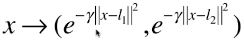的映射。

高斯核本质是在衡量样本和样本之间的“相似度”，在一个刻画“相似度”的空间中，让同类样本更好的聚在一起，进而线性可分。
疑问：
“衡量”的手段，经过这种映射之后，为什么同类样本能更好的分布在一起？

2）定义方式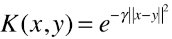；
x、y：样本或向量；
γ：超参数；高斯核函数唯一的超参数；
|| x - y ||：表示向量的范数，可以理解为向量的模；
表示两个向量之间的关系，结果为一个具体值；
高斯核函数的定义公式就是进行点乘的计算公式；
3）功能

先将原始的数据点（x， y）映射为新的样本（x'，y'）；
再将新的特征向量点乘（x' . y'），返回其点乘结果；
计算点积的原因：此处只针对 SVM 中的应用，在其它算法中的应用不一定需要计算点积；4）特点

高斯核运行开销耗时较大，训练时间较长；
一般使用场景：数据集 (m, n)，m < n；
一般应用领域：自然语言处理；
自然语言处理：通常会构建非常高维的特征空间，但有时候样本数量并不多；

5）高斯函数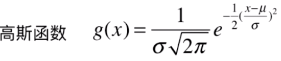正态分布就是一个高斯函数；
高斯函数和高斯核函数，形式类似；

6）其它

高斯核函数，也称为 RBF 核（Radial Basis Function Kernel），也称为径向基函数；
高斯核函数的本质：将每一个样本点映射到一个无穷维的特征空间；
无穷维：将 m*n 的数据集，映射为 m*m 的数据集，m 表示样本个数，n 表示原始样本特征种类，样本个数是无穷的，因此，得到的新的数据集的样本也是无穷维的；
高斯核升维的本质，使得线性不可分的数据线性可分；

三、RBF 转化特征数据原理

1）转化原理x：需要改变维度的样本；
np.array([l1, l2, ..., lm]) == X == np.array([x1, x2, ... , xm])：Landmark，地标，一般直接选取数据集 X 的所有样本作为地标；（共 m 个）
对于 (m, n) 的数据集：转化为 (m, m)  的数据集；将 n 维的样本转化为 m 维的样本；
对于原始数据集中的每一个样本 x，也可以有几个地标点，就将 x 转化为几维；

2）主要为两部分

先将原始的数据点映射为一种新的特征向量，再将新的特征向量点乘，返回其点乘结果；

维度转化：样本 x1 转化 x1' ：(e-γ||x1 - x1||**2, e-γ||x1 - x2||**2, e-γ||x1 - x3||**2, ..., e-γ||x1 - xm||**2)，同理样本 x2 的转化 x2' ；（地标点就是数据集 X 的样本点）
点乘计算：x1' . x2' == K(x1, x2) == e-γ||x1 - x2||**2，最终结果为一个具体值；

3）实例模拟维度转化过程

一维升到二维
原始样本分布：第一步：选取地标点：L1、L2 ；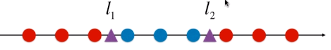第二步：升维计算四、程序模拟

目的：将线性不可分的数据变为线性可分；
方法：一维数据升到二维；
1）模拟数据集

x 数据集：每一个样本只有一个特征，且分布规律线性不可分；
np.arange(m, n, l)：将区间 [m, n) 按间距为 l 等分，等分后的数据点包含 m 值，不包含 n；
*len(x[y==0])： 是一个 list，list * C 表示将列表复制 C 份；
如：*5 == [0, 0, 0, 0, 0]import numpy as np
import matplotlib.pyplot as plt

x = np.arange(-4, 5, 1)
y = np.array((x >= -2) & (x <= 2), dtype='int')

plt.scatter(x[y==0], *len(x[y==0]))
plt.scatter(x[y==1], *len(x[y==1]))
plt.show()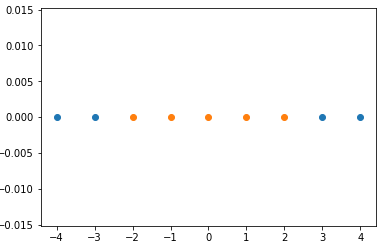2）经过高斯核，得到新的数据集

np.exp(m)：表示 e 的 m 次幂；
np.empty(元组)：（元组）=(m, n)，生成一个 m 行 n 列的空的矩阵；
enumerate(iterator)：返回可迭代对象的 index 和 value；
for i, data in enumerate(x)：i 存放向量 x 的 index，data 存放向量 x 的 index 对应的元素值；def gaussian(x, l):
# 此处直接将超参数 γ 设定为 1.0；
# 此处 x 表示一维的样本，也就是一个具体的值，l 相应的也是一个具体的数，因为 l 和 x 一样，从特征空间中选定；
gamma = 1.0
# 此处因为 x 和 l 都只是一个数，不需要再计算模，可以直接平方；
return np.exp(-gamma * (x-l)**2)

# 设定地标 l1、l2 为 -1和1
l1, l2 = -1, 1
x_new = np.empty((len(x), 2))

for i, data in enumerate(x):
x_new[i, 0] = gaussian(data, l1)
x_new[i, 1] = gaussian(data, l2)

plt.scatter(x_new[y==0, 0], x_new[y==0, 1])
plt.scatter(x_new[y==1, 0], x_new[y==1, 1])
plt.show()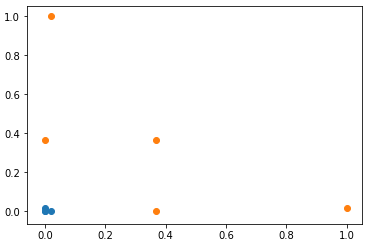展开全文SVM 机器学习
• 将2D高斯核函数根据非正交方向进行分解，得到一维的x轴和其他方向的高斯函数，通过统计学方法，将分解的高斯函数与卷积滤波器或者迭代滤波器结合进行边缘检测。
• ## 高斯核函数简介

千次阅读 2019-05-04 20:06:17
所谓径向基函数 (Radial Basis Function 简称 RBF), 就是某种沿径向对称的标量函数。 通常定义为空间中任一点...最常用的径向基函数是高斯核函数 ,形式为 k(||x-xc||)=exp{- ||x-xc||2/(2*σ2) } 其中xc为核函数中心...
• 高斯核函数 高斯核函数 (Gaussian kernel)，也称径向基 (RBF) 函数，用于将有限维数据映射到高维空间。通常定义为空间中任意一点 xxx 到某一中心点 x′x'x′ 之间的欧式距离的单调函数。 高斯核函数定义： k(x,x′)=...
• sklearn中的高斯核函数   这篇博客主要应用 sklearn 中封装的高斯核函数进行具体的分类。   我们还是先来看看高斯核函数里面 γγγ 的意思。之前我们说过高斯核函数与正态分布的式子很类似：      高斯...
•python opencv
• 支持向量机，高斯核函数背景介绍核心思想公式推导及主要函数核函数核心思想公式推导及函数名词介绍希尔伯特空间（Hilbert space）内积归一化和标准化内积空间（pre-Hilbert space/欧几里得空间）范数范数和距离...机器学习 支持向量机 深度学习
• 本人初看高斯核函数，写了一个小的Matlab程序，根据看的论文讲宽度参数设为了0.05，结果无论数据矩阵如何变化，得到的高斯核函数都是一个单位矩阵，非常不解，菜鸟一枚，求赐教
• 高斯核函数（Gaussian kernel），也称径向基 (RBF) 函数，是常用的一种核函数。它可以将有限维数据映射到高维空间，我们来看一下高斯核函数的定义： k(x,x′)=e−∣∣x−x′∣∣22δ2k(x,x')=e^{-\frac{||x-x'||^2}{......